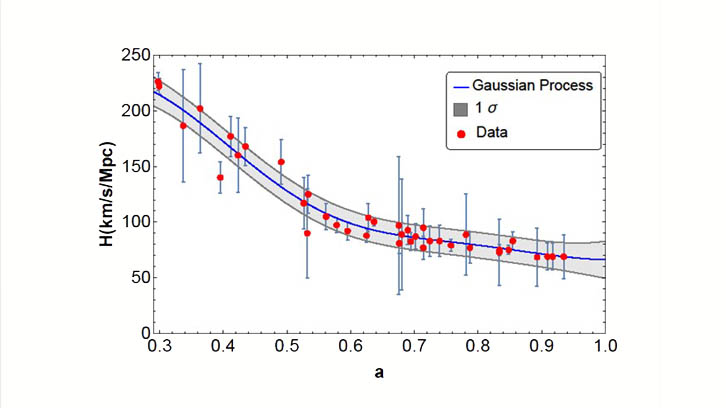• UABDivulga
17/10/2019

Does the Second Law Hold at Cosmic Scales?Observational data of the evolution of the Hubble factor and statistical approximation to the average evolution.

Thermodynamics, the branch of physics that regulates the interchange of energy between bodies due to their temperature difference, rests on three laws that resulted from the study of the behavior of systems in which gravity plays no role. These laws, especially the second law (the so-called, entropy law), govern the evolution of systems toward the thermodynamic equilibrium. After reaching it, the system remains in it (its temperature, pressure, etc, do not vary) so long as it goes unperturbed from the outside. According to this law the entropy of any isolated system does not diminish in the course of time.

In systems dominated by gravity, such as black holes, the laws of thermodynamics (as shown by Hawking, Bekenstein and Israel) still stand. This seems also true for larger systems as galaxies and clusters thereof. However, though these scales are huge compared with terrestrial standards they are only of astrophysical size, not cosmological. Thus one wonders whether they also hold at large scales, that is to say, at scales of the Universe. In reality we do not know how large the Universe might be.  We must content ourselves with dealing with sizes no larger than the distance traveled by light since the Universe began its expansion. This distance, known as the radius of the Hubble horizon, is the inverse of the present rate of cosmic expansion multiplied by the speed of light. Since we cannot gain information of what is going on beyond the horizon (no signal can travel faster than light) the area of the horizon is a measure of the entropy of the visible Universe (of that part of the Universe from which we can gather information).

Our work tries to answer the point raised above by recalling that the horizon has an entropy proportional to its area and that, in its turn, is proportional to the square of the inverse of the rate of expansion of the Universe (to the square of the inverse of the so-called Hubble factor). We used 39 measurements of the latter at different epochs, obtained by various researchers, ranging from the epoch when the visible Universe was about one third of its current size till the present. These data show that the entropy of the horizon increases as the Universe expands and that it tends to a constant value in the long run (in the far future). Since the entropy of the horizon is many orders of magnitude larger than the total entropy of all objects (galaxies, black holes, cosmic radiation, and so on) inside the horizon, we can assert that the Universe tends to a state of equilibrium (technically, to a state of maximum entropy) and therefore that it satisfies the entropy law. Put in another way, we can say that the second law of thermodynamics seems to hold at cosmic scales as well.

Diego Pavón
Area of ​​Condensed Matter Physics
Department of Physics
Universitat Autònoma de Barcelona

References

Gonzalez-Espinoza, Manuel & Pavon, Diego. (2019). Does the second law hold at cosmic scales? Monthly Notices of the Royal Astronomical Society, vol. 484, pp 2924-2930. DOI: 201910.1093/mnras/stz188.

View low-bandwidth version